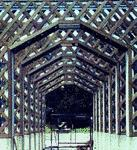# lattice

(redirected from latticing)
Also found in: Dictionary, Thesaurus, Medical.
Related to latticing: lattice

## lattice

1. an open framework of strips of wood, metal, etc., arranged to form an ornamental pattern
2.
a. a gate, screen, etc., formed of such a framework
b. (as modifier): a lattice window
3. something, such as a decorative or heraldic device, resembling such a framework
4. an array of objects or points in a periodic pattern in two or three dimensions, esp an array of atoms, ions, etc., in a crystal or an array of points indicating their positions in space

## Lattice

A network of bars, straps, rods, or laths crossing over and under one another; the result is a rectangular or diagonal checkered pattern, which may be varied by the width of the bands and the spacing of the members## Lattice

an algebraic concept. A lattice is a nonempty set S with two binary operations, join and meet, denoted by ⋃ and ⋂, respectively. In other words, to each pair of elements a and b in 5 there is associated a unique element ab in S, their join, and a unique element ab in 5, their meet. The operations satisfy the following axioms:

 (1) associativity (a ⋃ b) ⋃ c = a ⋃ (b ⋃ c) (a ⋂ b) ⋂ c = a ⋂ (b ⋂ c) (2) commutativity a ⋃ b = b ⋃ a a ⋂ b = b ⋂ a (3) absorption (a ⋃ b) ⋂ a = a (a ⋂ b) ⋃ a = a

Examples of lattices include the set of positive integers with ab the greatest common divisor and ab the least common multiple of a and b, the set of all subsets of an arbitrary set with set theoretic union and intersection as the lattice operations, and the set of real numbers with ab = max (a, b) and ab = min (a, b).

Certain lattices satisfying additional requirements have been studied in great detail. Examples of such lattices are distributive lattices, modular (or Dedekind) lattices, and complemented lattices. A very important type of lattice is a Boolean algebra, that is, a distributive lattice with zero and one in which each element has a complement. Boolean algebras play an important role in mathematical logic and probability theory. Other kinds of lattices are used in set theory, topology, and functional analysis.

There is a natural way of using the lattice operations to partially order the lattice and thus establish the equivalence of lattice theory and the theory of partially ordered sets.

The concept of the lattice first appeared in the mid-19th century and was first fully defined by J. W. R. Dedekind.

### REFERENCES

Birkhoff, G. Teoriia struktur. Moscow, 1952. (Translated from English.)
Skorniakov, L. A. Elementy teorii struktur. Moscow, 1970.
Sikorski, R. Bulevy algebry. Moscow, 1969. (Translated from English.)
Vladimirov, D. A. Bulevy algebry. Moscow, 1969.

## lattice

(civil engineering)
A network of crisscrossed strips of metal or wood.
(crystallography)
A regular periodic arrangement of points in three-dimensional space; it consists of all those points P for which the vector from a given fixed point to P has the form n1a+ n2b+ n3c, where n1, n2, and n3 are integers, anda, b,andcare fixed, linearly independent vectors. Also known as periodic lattice; space lattice.
(mathematics)
A partially ordered set in which each pair of elements has both a greatest lower bound and least upper bound.
A pattern formed by two or more families of intersecting lines of position, such as the hyperbolic lines of position from two or more loran stations.
(nucleonics)
An orderly array or pattern of nuclear fuel elements and moderator in a reactor or critical assembly.

## lattice

1. A network, often diagonal, of strips, rods, bars, laths, or straps of metal or wood, used as screening or for airy, ornamental constructions.
2. A regular member triangularly braced, e.g., a lattice girder, a lattice truss.

## lattice

A network of intersecting positional lines printed on a map or chart from which a fix may be obtained. See hyperbolic navigation system.

## lattice

(theory)
A partially ordered set in which all finite subsets have a least upper bound and greatest lower bound.

This definition has been standard at least since the 1930s and probably since Dedekind worked on lattice theory in the 19th century; though he may not have used that name.